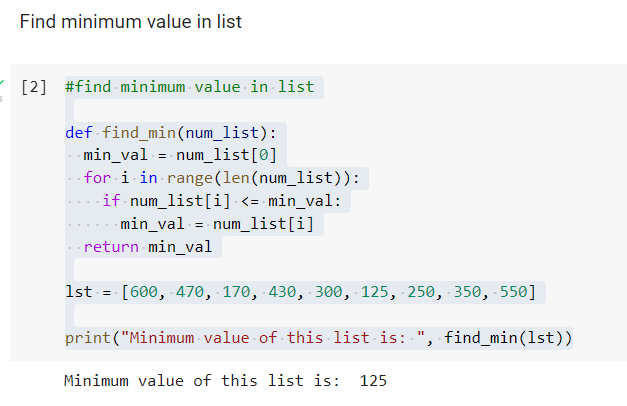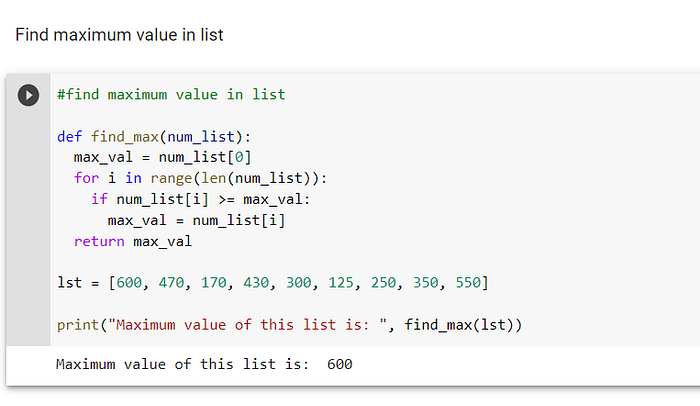# How to Find Minimum and Maximum Values in a List Using Python

## A tutorial on finding minimum and maximum values in a list using Python.In previous posts, I have been covering statistical measurements, so in this post, I have decided to discuss two methods from what is called the five number summary. We have already gone over one measure from the five-number summary, which is the mean, so now I intend to look into the minimum and maximum statistical values. Although there are functions in Python that will calculate these values automatically, it is a good idea to know the algorithms so you can create your own functions if necessary.

The pseudocode for computing the minimum is as follows:

1. Define the function, find_min, which accepts a list as input.
2. Define the variable, min_val, which has the first element of the list posited in it.
3. Create a for loop, which iterates through the length of the list.
4. If the element in the list is less than min_val then min_val becomes the value of that element.
5. When the for loop has completed its iterations, the function will return the variable, min_val.The only thing that is necessary to find the maximum value of the list is a slight modification of the find_min function. The pseudocode for this function is listed below:-

1. Define the function, max_num, which accepts a list as input.
2. Define the variable, max_val, which has the first element of the list posited in it.
3. Create a for loop, which iterates through the length of the list.
4. If the element in the list is more than max_val then max_val becomes the value of that element.
5. When the for loop has completed its iterations, the function will return the variable, max_val.The two functions above are critical to statistics, as is the mean. Now, in order to complete the discussion of the five-number summary, we will cover percentiles in an upcoming post.

I have created a code review for this post, which can be viewed here: https://www.youtube.com/watch?v=8MiR_j6W0Pg